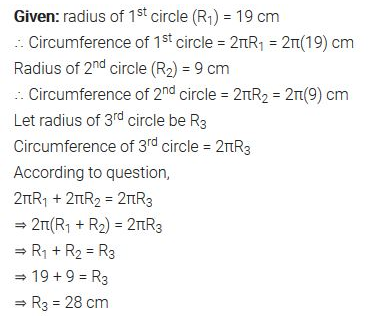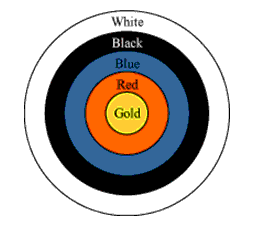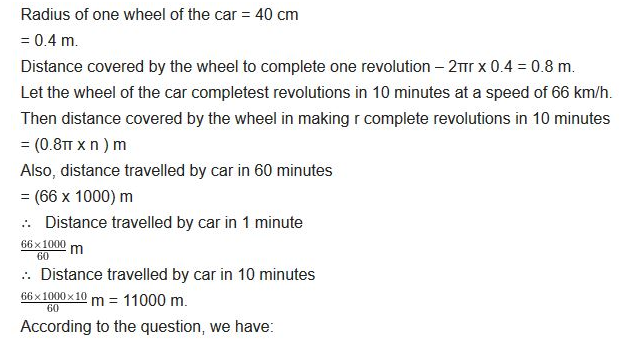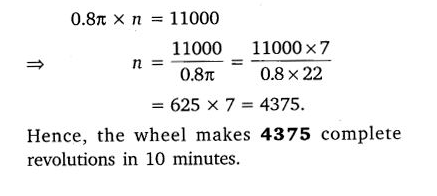• +91 9971497814
• info@interviewmaterial.com

# Areas Related to Circles Ex-12.1 Interview Questions Answers

### Related Subjects

Question 1 :

The radii of twocircles are 19 cm and 9 cm respectively. Find the radius of the circle whichhas a circumference equal to the sum of the circumferences of the two circles.

Answer 1 :Question 2 :

The radii of twocircles are 8 cm and 6 cm, respectively. Find the radius of the circle havingarea equal to the sum of the areas of the two circles.

Radius of 1st circle = 8 cm(given)

Area of 1st circle = π(8)2 =64π

Radius of 2nd circle = 6 cm(given)

Area of 2nd circle = π(6)2 =36π

So,

The sum of 1st and 2nd circlewill be = 64π+36π = 100π

Now, assume that the radius of 3rd circle= R

Area of the circle 3rd circle = πR2

It is given that the area of the circle 3rd circle= Area of 1st circle + Area of 2nd circle

Or, πR2 = 100πcm2

R2 = 100cm2

So, R = 10cm

Question 3 :

Fig. 12.3 depicts anarchery target marked with its five scoring regions from the centre outwards asGold, Red, Blue, Black and White.

Answer 3 : The diameter of the region representing Gold score is 21 cm and each of the other bands is 10.5 cm wide. Find the area of each of the five scoring regions.Solution:

The radius of 1st circle, r1 =21/2 cm (as diameter D is given as 21 cm)

So, area of gold region = π r1=π(10.5)= 346.5 cm2

Now, it is given that each of the other bandsis 10.5 cm wide,

So, the radius of 2nd circle,r2 = 10.5cm+10.5cm = 21 cm

Thus,

Area of red region = Area of 2nd circle − Areaof gold region = (πr22−346.5) cm2

= (π(21)2 − 346.5) cm2

= 1386 − 346.5

= 1039.5 cm2

Similarly,

The radius of 3rd circle, r3 =21 cm+10.5 cm = 31.5 cm

The radius of 4th circle, r4 =31.5 cm+10.5 cm = 42 cm

The Radius of 5th circle, r5 =42 cm+10.5 cm = 52.5 cm

For the area of nth region,

A = Area of circle n – Area of circle (n-1)

Area of blue region (n=3) = Area of third circle – Area ofsecond circle

= π(31.5)2 – 1386 cm2

= 3118.5 – 1386 cm2

= 1732.5 cm2

Area of black region (n=4) = Area of fourth circle – Area ofthird circle

= π(42)2 – 1386 cm2

= 5544 – 3118.5 cm2

= 2425.5 cm2

Area of white region (n=5) = Area of fifth circle – Area offourth circle

= π(52.5)2 – 5544 cm2

= 8662.5 – 5544 cm2

= 3118.5 cm2

Question 4 :

The wheels of a carare of diameter 80 cm each. How many complete revolutions does each wheel makein 10 minutes when the car is travelling at a speed of 66 km per hour?

Answer 4 :Question 5 :

Tick the correctSolution: in the following and justify your choice : If the perimeter and thearea of a circle are numerically equal,

then the radius of the circle is

(A) 2 units               (B) π units

(C) 4 units               (D) 7 units

Solution:

Since the perimeter of the circle = area ofthe circle,

2πr = πr2

Or, r = 2

So, option (A) is correct i.e. the radius ofthe circle is 2 units.

Todays Deals### Areas Related to Circles Ex-12.1 Contributorskrishan

Name:
Email:

# Latest News# 9000 interview questions in different categories